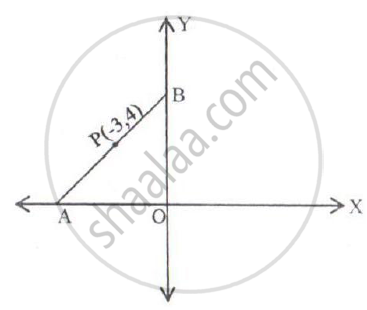# In the Figure Given Below, the Line Segment Ab Meets X-axis at a and Y-axis at B. the Point P(-3, 4) on Ab Divides It in the Ratio 2:3. Find the Coordinates of a and B. - Mathematics

#### Question

In the figure given below, the line segment AB meets X-axis at A and Y-axis at B. The point P(-3, 4) on AB divides it in the ratio 2:3. Find the coordinates of A and B.#### Solution

Let the coordinates of A and B be (x, 0) and (0, y) respectively.

Given P divides AB is the ratio 2:3,

Using section formula, we have

-3 = (2 xx 0 + 3 xx x)/(2 + 3)

-3 = "3x"/5

-15 = 3x

x = -5

and

4 = (2 xx y + 3 xx 0)/(2 + 0)

4 = "2y"/5

20 = 2y

y = 10

Thus, the coordinates of A and B are (–5, 0) and (0, 10) respectively.

Is there an error in this question or solution?